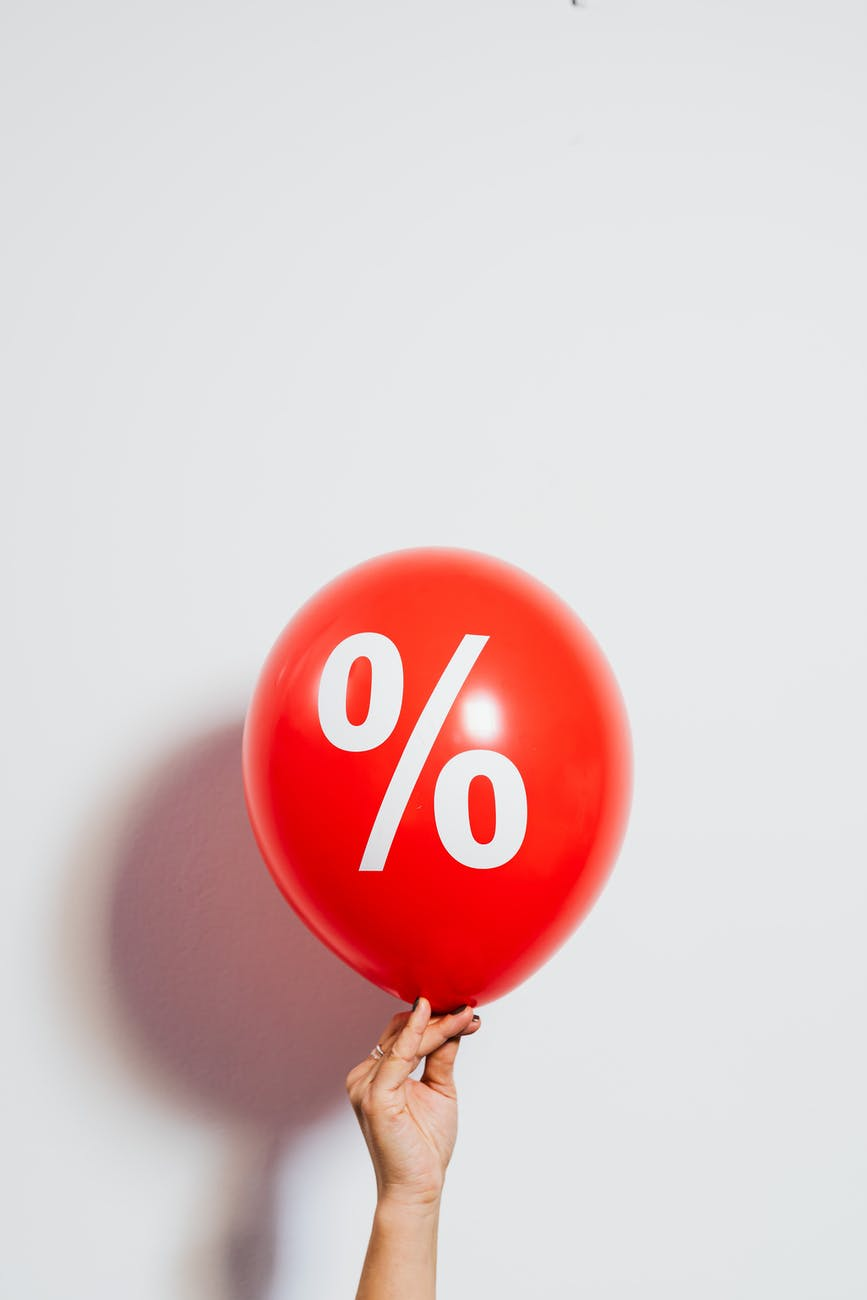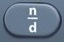# Simple InterestBasics

The formula provided for Interest (aka Simple Interest) by the Mathematics Formula Sheet is straightforward, but it can be rearranged to ask questions about principal, rate, and time, too.

A question involving Interest is often a plug-in (where you end up plugging in numbers for letters).

Interest = principal × rate × time
I = prt
I = Interest = additional amount of money owed (on a loan) or earned (on a deposit)
p = principal = original amount of money owed or deposited

r = rate = percentage charged for interest
t = time = period of time over which interest is charged

Formulas (Mathematics Formula Sheet)
I = prt

Formulas (NOT on Mathematics Formula Sheet)

p =$\bf\displaystyle\frac{I}{rt}$

r =$\bf\displaystyle\frac{I}{pt}$

t =$\bf\displaystyle\frac{I}{pr}$

Question
A loan of $1000.00 is taken out at a rate of 7% for two years. How much interest is charged over this time? Answer$140.00

I=prt
I = 1000 × 7% × 2 = 140

Input Display Commentblinker clears screen
1000 × 7× 2 1000*7%*2 I = prt140 Answer
###### Simple Interest

Question
$5000.00 is deposited in a bank at a rate of 3% for six years. How much interest is earned over this time? Answer$900.00

I = prt
I = 5000 × 3% × 6 = 900

Input Display Commentblinker clears screen
5000 × 3× 6 5000*3%*6 I = prt900 Answer
###### Simple Interest

Question

$105.00 in interest was earned on a 5-year certificate of deposit at a rate of 3%. What was the initial deposit? Answer$700.00

p =$\bf\displaystyle\frac{I}{rt}$

p =$\bf\displaystyle\frac{105}{3\% x 5}$ = 700

Input Display Commentblinker clears screen105× 5$\bf\displaystyle\frac{105}{3\%*5}$ p =$\bf\displaystyle\frac{I}{rt}$700 Answer
###### Simple Interest

Question

$310.00 in interest was earned on a 5-year certificate of deposit with an initial deposit of$4000.00.  What was the interest rate in decimal terms?

0.0155

r =$\bf\displaystyle\frac{I}{pt}$

r =$\bf\displaystyle\frac{310}{4000 x 5}$ = 0.0155

Input Display Commentblinker clears screen3104000 × 5$\bf\displaystyle\frac{310}{4000*5}$ r =$\bf\displaystyle\frac{I}{pt}$0.0155 Answer
###### Simple Interest

Question
With reference to the above question, what was the interest rate in percent terms?

1.55%

See Percent.
To convert a decimal to percent, move the decimal point two places to the right.
0.0155 = 1.55%

Question

$320.00 in interest was earned on an initial deposit of$2000.00 at a rate of 4%.  How long was the money earning interest?

4 years

t =$\bf\displaystyle\frac{I}{pr}$

t =$\bf\displaystyle\frac{320}{2000 x 4\%}$ = 4

Input Display Commentblinker clears screen3202000 × 4$\bf\displaystyle\frac{320}{2000*4\%}$ t =$\bf\displaystyle\frac{I}{pr}$4 Answer
###### Simple Interest

Practice – Questions

1.  What is the interest owed on a car loan of $25000.00 at a rate of 3% for five years? 2. What is the interest owed on a credit card balance of$5000.00 at a rate of 20% for five years?

3.  $215.00 in interest was earned on a 4-year certificate of deposit at a rate of 5%. What was the initial deposit? 4.$3122.00 in interest was paid on a credit card balance of $4000.00 over five years. What was the interest rate in percent terms? 5.$800.00 in interest was earned on an initial deposit of $2000.00 at a rate of 5%. How long was the money earning interest? Practice – Answers 1.$3750.00

2.  $5000.00 3.$1075.00

4.  15.61%

5.  8 years